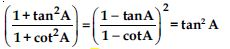# NCERT Solutions for Class 10 Math Chapter 8 - Introduction to Trigonometry

Find NCERT Solutions at Aasoka which is beneficial for students as it helps them in getting a good grasp on the concepts. The questions which are framed by the experts are as per the latest syllabus and their guidelines. The NCERT Solutions for Class 10th make students familiar with the exam pattern. They learn how to write the answers as per the given marking scheme. With regular practice, students can solve complex problems without any hassle.

The chapter “Trigonometry” provides basic knowledge which includes the ratio of right triangles, a few trigonometric identities, calculation of trigonometric ratios for the given angles, and so much more. Students can learn formulas of trigonometry so that they can have it on the tips of their hands in times of need. These formulas will benefit them if they choose to take Math in their Class 11th and 12th.

##### Question 1:

In ΔABC, right-angled at B, AB = 24 cm;
BC = 7 cm. Determine:
(i) sin A, cos A (ii) sin C, cos C

Given that,
AB = 24 cm; BC = 7 cm; $\angle$B = 90$°$
By using Pythagoras Theorem,
AC2 = AB2 + BC2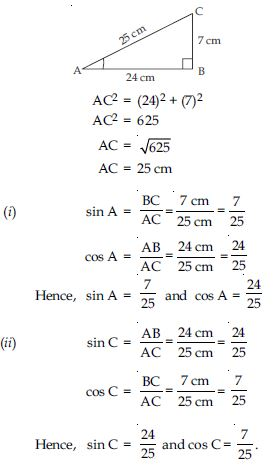##### Question 2: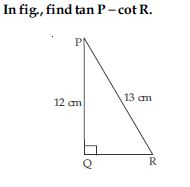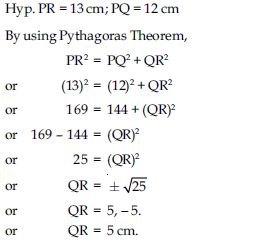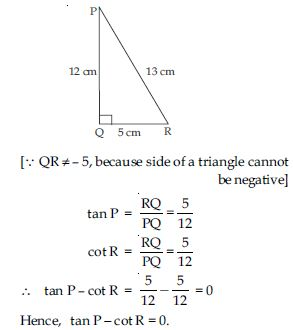##### Question 3: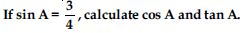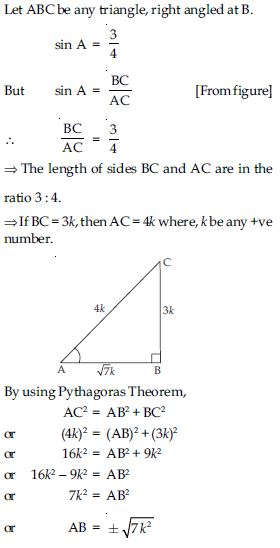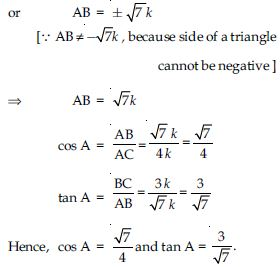##### Question 4:

Given 15 cot A = 8, find sin A and sec A.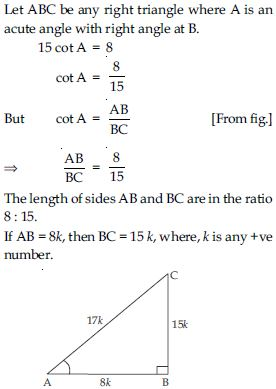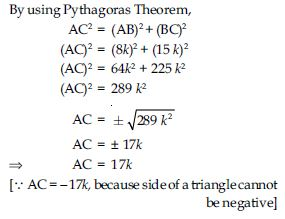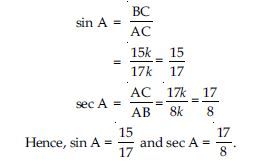##### Question 5: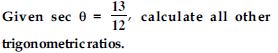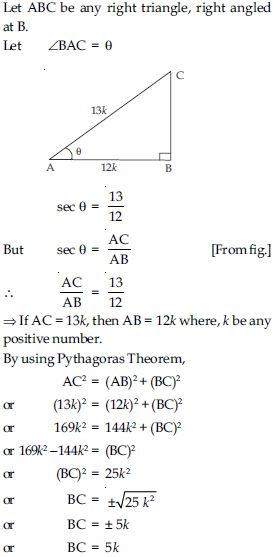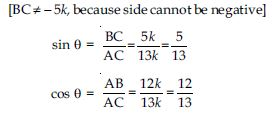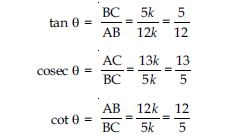##### Question 6:

If $\angle$A and $\angle$B are acute angles such that cos A = cos B, then show that: $\angle$A = $\angle$B.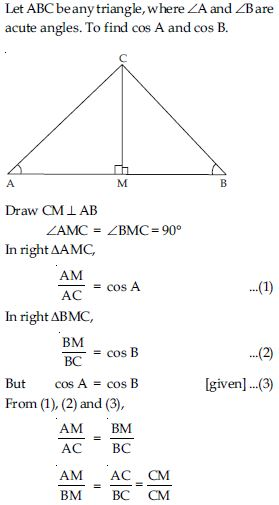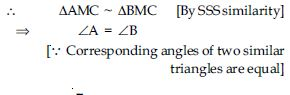##### Question 7: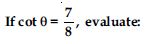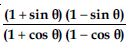$\angle$ABC = $\mathrm{\theta }$.
In right triangle ABC, right angled at C.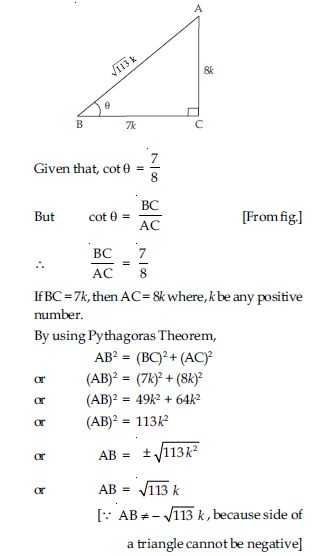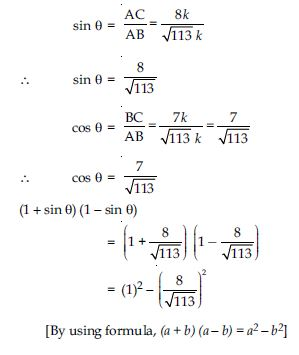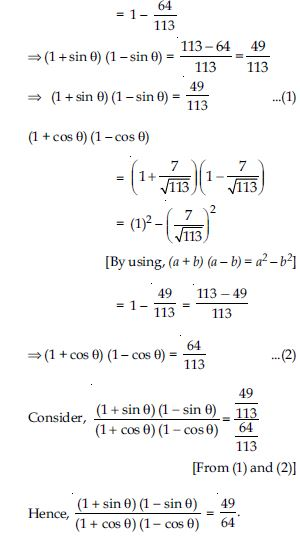##### Question 8:cot2 $\mathrm{\theta }$.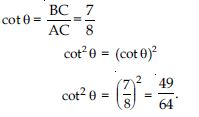##### Question 9: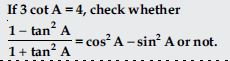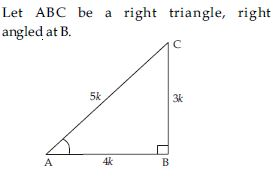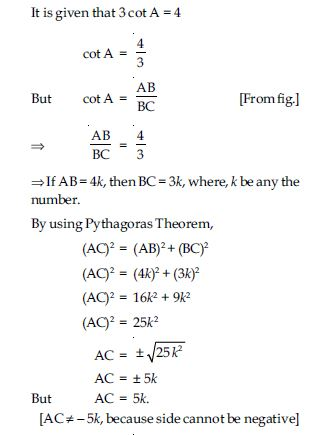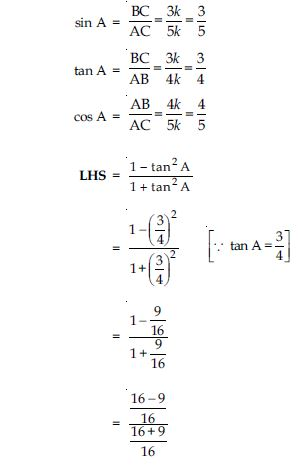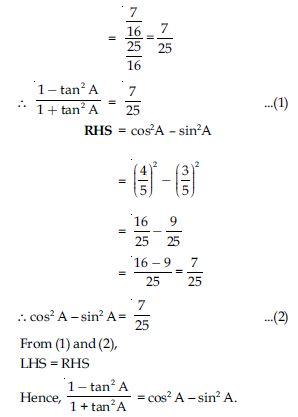##### Question 10: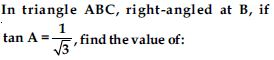sin A cos C + cos A sin C

Given: ΔABC with right angled at B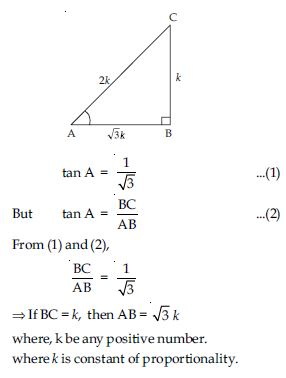In right triangle ABC,
By using Pythagoras Theorem,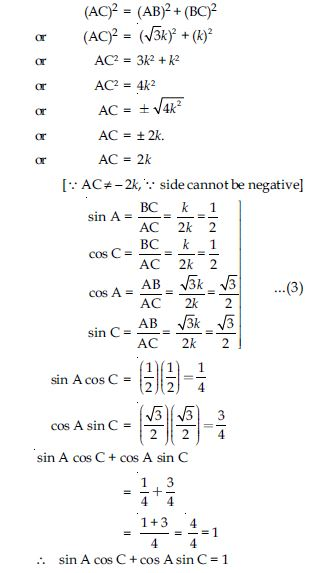##### Question 11:cos A cos C - sin A sin C.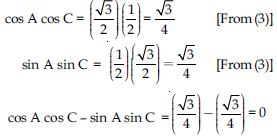##### Question 12:

In $∆$PQR, right-angled at Q, PR + QR = 25 cm and PQ = 5 cm. Determine the values of sin P, cos P and tan P.

Given: ΔPQR, right angled at Q
PR + QR = 25 cm
PQ = 5 cm
In right triangle PQR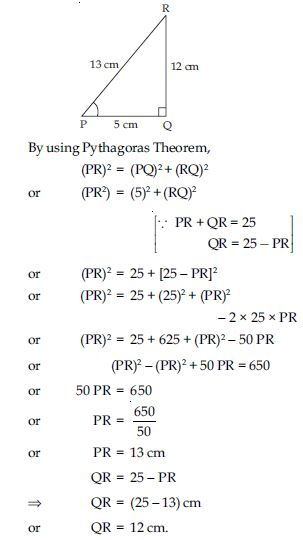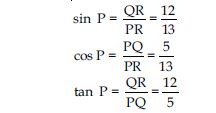##### Question 13:

The value of tan A is always less than 1.

FALSE

##### Question 14: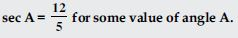TRUE

##### Question 15:

cos A is abbreviation used for the cosecant of angle A.

FALSE

##### Question 16:

cot A is the product of cot and A.

FALSE

##### Question 17: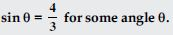FALSE

##### Question 18:

Evaluate the following:
sin 60$°$ cos 30$°$ + sin 30$°$ cos 60$°$##### Question 19:

Evaluate the following:
2 tan2 45$°$ + cos2 30$°$ - sin2 60$°$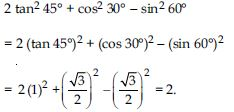##### Question 20:

Evaluate the following: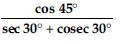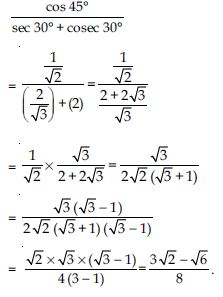##### Question 21:

Evaluate the following: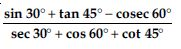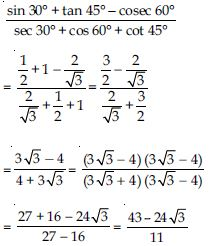##### Question 22:

Evaluate the following: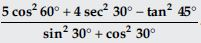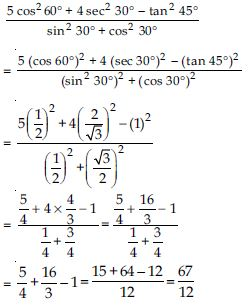##### Question 23: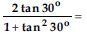1. sin 60$°$
2. cos 60$°$
3. tan 60$°$
4. sin 30$°$

(i) sin 60$°$

##### Question 24:1. tan 90$°$
2. 1
3. sin 45$°$
4. 0

(iv) 0

##### Question 25:

sin 2A = 2 sin A is true when A =

1. 0$°$
2. 30$°$
3. 45$°$
4. 60$°$

(i) 0$°$

##### Question 26: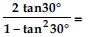1. cos 60$°$
2. sin 60$°$
3. tan 60$°$
4. sin 30$°$

(iii) tan 60$°$

##### Question 27: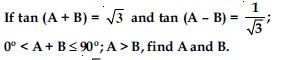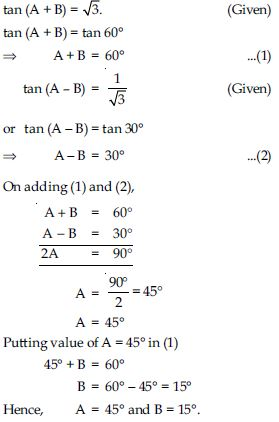##### Question 28:

sin (A + B) = sin A + sin B.

FALSE

##### Question 29:

The value of sin $\mathrm{\theta }$ increases as $\mathrm{\theta }$ increases.

TRUE

##### Question 30:

The value of cos $\mathrm{\theta }$ increases as $\mathrm{\theta }$ increases.

FALSE

##### Question 31:

sin $\mathrm{\theta }$ = cos $\mathrm{\theta }$ for all value of $\mathrm{\theta }$.

FALSE

##### Question 32:

cot A is not defined for A = 0$°$.

TRUE

##### Question 33:

Evaluate: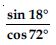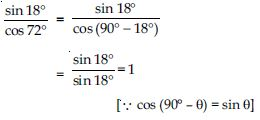##### Question 34:

Evaluate: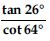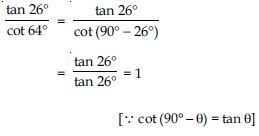##### Question 35:

Evaluate:
cos 48$°$ - sin 42$°$##### Question 36:

Evaluate:
cosec 31$°$ - sec 59$°$.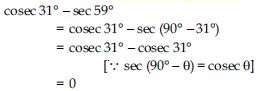##### Question 37:

Show that:
tan 48$°$ tan 23$°$ tan 42$°$ tan 67$°$ = 1

LHS
= tan 48$°$ tan 23$°$ tan 42$°$ tan 67$°$
= tan 48$°$ × tan 23$°$ ×
tan (90$°$ - 48$°$) × tan (90$°$ - 23$°$)
= tan 48$°$ × tan 23$°$ × cot 48$°$ × cot 23$°$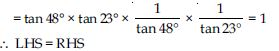##### Question 38:

Show that:
cos 38$°$ cos 52$°$ - sin 38$°$ sin 52$°$ = 0

LHS = cos 38$°$ cos 52$°$ - sin 38$°$ sin 52$°$
= cos 38$°$ × cos (90$°$ - 38$°$)
- sin 38$°$ × sin (90$°$ - 38$°$)
= cos 38$°$ × sin 38$°$ - sin 38$°$ × cos 38$°$
= 0.
∴ LHS = RHS

##### Question 39:

If tan 2A = cot (A - 18$°$), where 2A is an acute angle, find the value of A.

Given: tan 2A = cot (A - 18$°$)
$⇒$ cot (90$°$ - 2A) = cot (A - 18$°$)
[∵ cot (90$°$ - $\mathrm{\theta }$) = tan $\mathrm{\theta }$]
$⇒$ 90$°$ - 2A = A - 18$°$
$⇒$ 3A = 108$°$
$⇒$ A = 36$°$.

##### Question 40:

If tan A = cot B, prove that: A + B = 90$°$.

Given that: tan A = cot B
$⇒$ tan A = tan (90$°$ - B)
[∵ tan (90$°$ - $\mathrm{\theta }$) = cot $\mathrm{\theta }$]
$⇒$ A = 90$°$ - B
$⇒$ A + B = 90$°$ [Hence proved]

##### Question 41:

If sec 4A = cosec (A - 20$°$), where 4A is an acute angle, find the value of A.

Given that: sec 4A = cosec (A - 20$°$) $⇒$ cosec (90$°$ - 4A) = cosec (A - 20$°$) [∵ cosec (90$°$ - $\mathrm{\theta }$) = sec $\mathrm{\theta }$] $⇒$ 90$°$ - 4A = A - 20$°$ $⇒$ 5A = 110$°$ $⇒$ A = 22$°$

##### Question 42: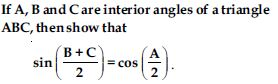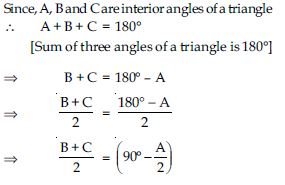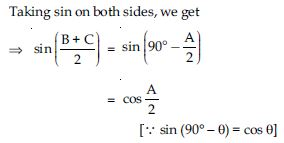##### Question 43:

Express sin 67$°$ + cos 75$°$ in terms of trigonometric ratios of angles between 0$°$ and 45$°$.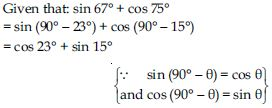##### Question 44:

Express the trigonometric ratios sin A, sec A and tan A in terms of cot A.

By using Identity,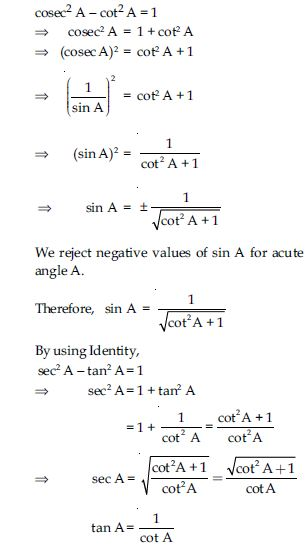##### Question 45:

Write all the other trigonometric ratios of $\angle$A in terms of sec A.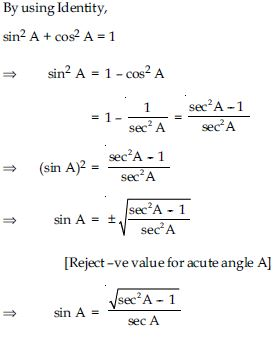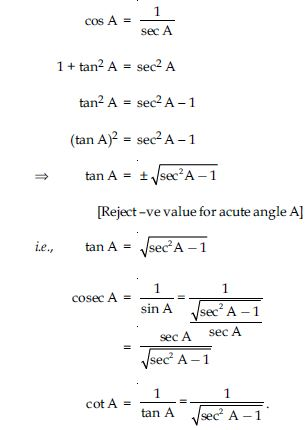##### Question 46:

Evaluate: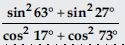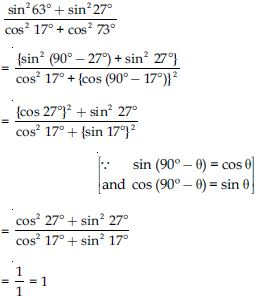##### Question 47:

Evaluate:
sin 25$°$ cos 65$°$ + cos 25$°$ sin 65$°$

sin 25$°$ cos 65$°$ + cos 25$°$ sin 65$°$
= sin 25$°$ × cos (90$°$ - 25$°$)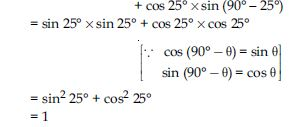##### Question 48:

9 sec2 A - 9 tan2 A =

1. 1
2. 9
3. 8
4. 0

(ii) 9

##### Question 49:

(1 + tan $\mathrm{\theta }$ + sec $\mathrm{\theta }$) (1 + cot $\mathrm{\theta }$ - cosec $\mathrm{\theta }$) =

1. 0
2. 1
3. 2
4. - 1

(iii) 2

##### Question 50:

(sec A + tan A) (1 - sin A) =

1. sec A
2. sin A
3. cosec A
4. cos A

(iv) cos A

##### Question 51: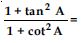1. sec2A
2. - 1
3. cot2A
4. tan2A

(iv) tan2A

##### Question 52:

Prove the following identities, where the angles involved are acute angles for which the expressions are defined.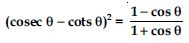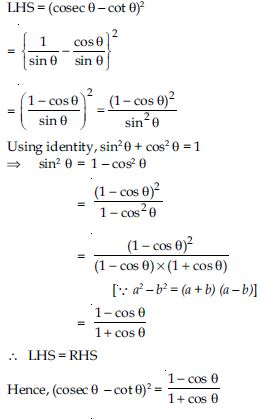##### Question 53:

Prove the following identities, where the angles involved are acute angles for which the expressions are defined.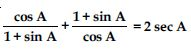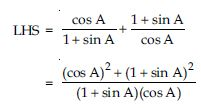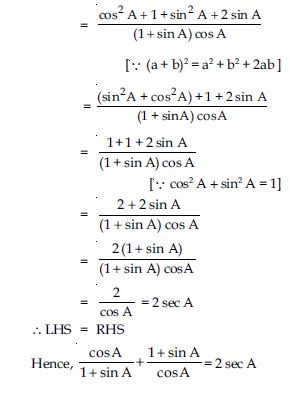##### Question 54:

Prove the following identities, where the angles involved are acute angles for which the expressions are defined.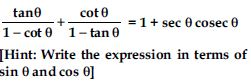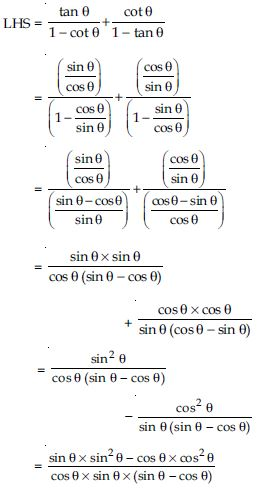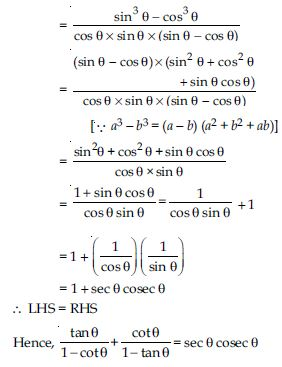##### Question 55:

Prove the following identities, where the angles involved are acute angles for which the expressions are defined.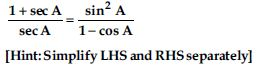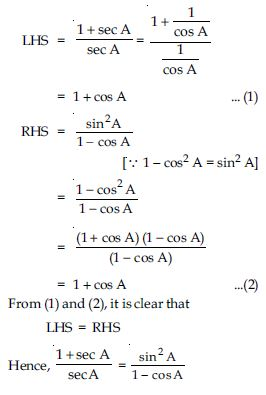##### Question 56:

Prove the following identities, where the angles involved are acute angles for which the expressions are defined.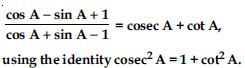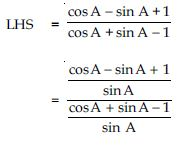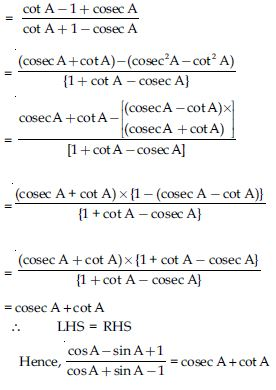##### Question 57:

Prove the following identities, where the angles involved are acute angles for which the expressions are defined.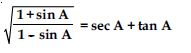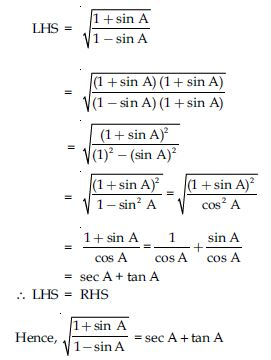##### Question 58:

Prove the following identities, where the angles involved are acute angles for which the expressions are defined.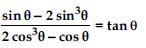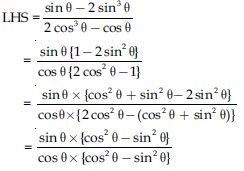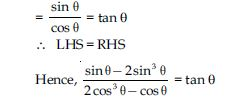##### Question 59:

Prove the following identities, where the angles involved are acute angles for which the expressions are defined.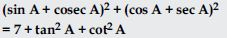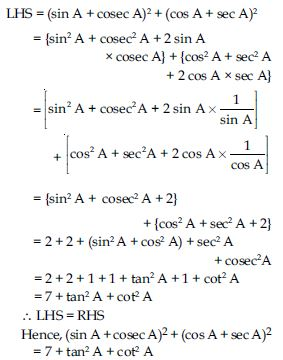##### Question 60:

Prove the following identities, where the angles involved are acute angles for which the expressions are defined.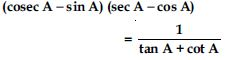[Hint: Simplify LHS and RHS separately]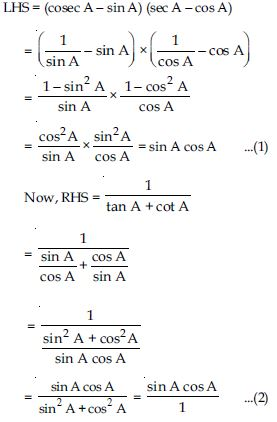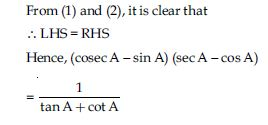##### Question 61:

Prove the following identities, where the angles involved are acute angles for which the expressions are defined.